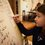# Computer Science Fundamentals Course: Treaps

A while back, I was faced the following issue (copied from my blog):

The problem (at https://brilliant.org/practice/treaps/?p=4):The Problem

Me working on it…My Solution

...The solution on Brilliant was different...Brilliant's SolutionBrilliant's Explanation

...However, only 1 page earlier, the explanation said...Note

Since then, Brilliant has given a solution to my question:

A) The reason why his selected answer is incorrect is because that tree violates the BST ordering invariant: the node containing 2 must appear in the left subtree of the root, because 2 < 5.

We gave this common misconception as an example in the previous page, saying You might ask, "Well, why can't the 40/4 node be drawn under the 70/10 node?" The answer is that although this would satisfy the max-heap property of the tree, it will violate the property that the keys must be sorted in the correct order (according to an "in order" traversal). I.e. all keys on the right branch of a node must be greater than or equal to the key of that node. In this example, 40/4 would violate this since 40 < 50.

B) The comment 1 page earlier says "Note: Because of the randomization that occurs in step one, this process is not deterministic. However, once step one has been completed, there is a unique way to construct the tree." The step one that is referenced is "Begin with all the priority values randomly sorted in an array.".

In the problem, step one has already been completed (since we've stated the set of priorities), hence there is a unique way to construct the tree.

However, that is my question! It's not replying the question, it's reasking (or, even worse, ignoring) the question!!Note by Simon Houben
2 years, 1 month ago

This discussion board is a place to discuss our Daily Challenges and the math and science related to those challenges. Explanations are more than just a solution — they should explain the steps and thinking strategies that you used to obtain the solution. Comments should further the discussion of math and science.

When posting on Brilliant:

• Use the emojis to react to an explanation, whether you're congratulating a job well done , or just really confused .
• Ask specific questions about the challenge or the steps in somebody's explanation. Well-posed questions can add a lot to the discussion, but posting "I don't understand!" doesn't help anyone.
• Try to contribute something new to the discussion, whether it is an extension, generalization or other idea related to the challenge.

MarkdownAppears as
*italics* or _italics_ italics
**bold** or __bold__ bold
- bulleted- list
• bulleted
• list
1. numbered2. list
1. numbered
2. list
Note: you must add a full line of space before and after lists for them to show up correctly
paragraph 1paragraph 2

paragraph 1

paragraph 2

[example link](https://brilliant.org)example link
> This is a quote
This is a quote
    # I indented these lines
# 4 spaces, and now they show
# up as a code block.

print "hello world"
# I indented these lines
# 4 spaces, and now they show
# up as a code block.

print "hello world"
MathAppears as
Remember to wrap math in $$ ... $$ or $ ... $ to ensure proper formatting.
2 \times 3 $2 \times 3$
2^{34} $2^{34}$
a_{i-1} $a_{i-1}$
\frac{2}{3} $\frac{2}{3}$
\sqrt{2} $\sqrt{2}$
\sum_{i=1}^3 $\sum_{i=1}^3$
\sin \theta $\sin \theta$
\boxed{123} $\boxed{123}$

Sort by:

Apologies for the late response.

In the solution that you have provided, the numbers in green are supposed to satisfy the BST property. The BST property says that if in any subtree, the root has the number $x$, then the number on any node on the subtree on the left should be $\leq x$ and on the right should be $\geq x$. Your tree violates this property because the node with the green 2 occurs on the right subtree, violating the BST property!

- 2 years, 1 month ago